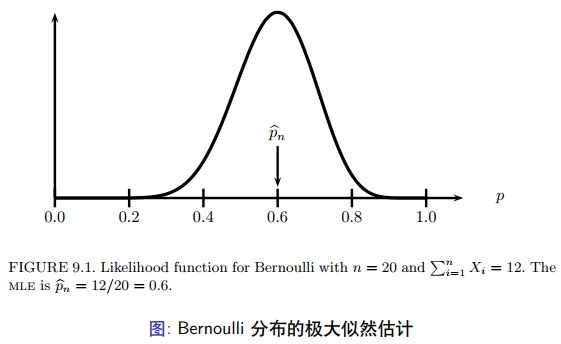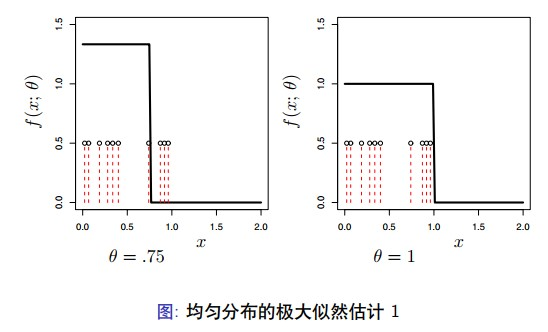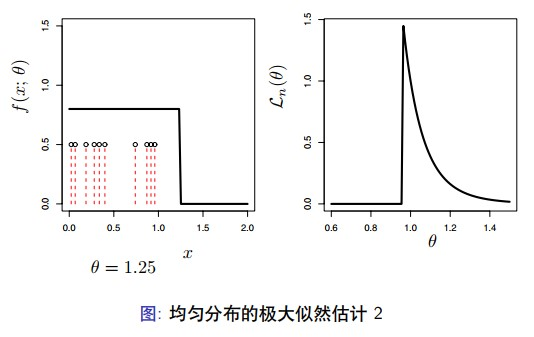$$\mathfrak{F}=\{f(x ; \theta): \theta \in \Theta\}$$

1. 有先验知识可以知道数据近似服从某种参数化模型。如，交通事故发 生的次数近似服从泊松分布。
2. 参数化模型的推断为理解非参数方法提供了背景知识。 这样，我们还是要学习参数化模型。

## 关注参数

\begin{aligned} \tau &=\mathbb{P}(X>1)=1-\mathbb{P}(X<1)=1-\mathbb{P}\left(\dfrac{X-\mu}{\sigma}<\dfrac{1-\mu}{\sigma}\right) \\ &=1-\mathbb{P}\left(Z<\dfrac{1-\mu}{\sigma}\right)=1-\Phi\left(\dfrac{1-\mu}{\sigma}\right) \end{aligned}

$$f(x ; \alpha, \beta)=\dfrac{1}{\beta^{\alpha} \Gamma(\alpha)} x^{\alpha-1} e^{-x / \beta}, \quad x>0$$

$$\Gamma(\alpha)=\int_{0}^{\infty} y^{\alpha-1} e^{-y} d y$$

$$T(\alpha, \beta)=\mathbb{E}_{\theta}\left(X_{1}\right)=\alpha \beta$$

## 矩估计

• 第一种用于参数估计的方法称为矩方法。我们将会看到，这些估计 器的并不是最优的，但是他们却容易计算。这些方法得到的结果对 于需要迭代数值计算的方法是很好的初始值。
• 假定我们有参数 $\theta=\left (\theta_{1}, \ldots, \theta_{k}\right)$ 包括 $k$ 个成分。对于 $1 \leq j \leq k$, 定义 $j$ 阶矩 (Moment) 为
$$\alpha_{j} \equiv \alpha_{j}(\theta)=\mathbb{E}_{\theta}\left(X^{j}\right)=\int x^{j} d F_{\theta}(x)$$
• 同时，定义第 $j$ 阶样本矩 (Sample Moment) 为
$$\widehat{\alpha}_{j}=\dfrac{1}{n} \sum_{i=1}^{n} X_{i}^{j}$$

$$\begin{array}{c} \alpha_{1}\left(\widehat{\theta}_{n}\right)=\widehat{\alpha}_{1} \\ \alpha_{2}\left(\widehat{\theta}_{n}\right)=\widehat{\alpha}_{2} \\ :: \\ \alpha_{k}\left(\widehat{\theta}_{n}\right)=\widehat{\alpha}_{k} \end{array}$$

$$\widehat{p}_{n}=\dfrac{1}{n} \sum_{i=1}^{n} X_{i}$$

1. 估计器 $\hat {\theta}_{n}$ 存在的概率趋近于 1 。
2. 这个估计器是一致估计，即 $\widehat {\theta}_{n} \stackrel {\mathrm {P}}{\longrightarrow} \theta$ 。
3. 这个估计是渐进正态的，即
$$\sqrt{n}\left(\widehat{\theta}_{n}-\theta\right) \rightsquigarrow N(0, \Sigma)$$

$$\Sigma=g \mathbb{E}_{\theta}\left(Y Y^{T}\right) g^{T}$$

$$Y=\left(X, X^{2}, \ldots, X^{k}\right)^{T}, g=\left(g_{1}, \ldots, g_{k}\right), g_{j}=\partial \alpha_{j}^{-1}(\theta) / \partial \theta$$

## 极大似然估计

$$\mathcal{L}_{n}(\theta)=\prod_{i=1}^{n} f\left(X_{i} ; \theta\right)$$

$$\mathcal{L}_{n}(p)=\prod_{i=1}^{n} f\left(X_{i} ; p\right)=\prod_{i=1}^{n} p^{X_{i}}(1-p)^{1-X_{i}}=p^{S}(1-p)^{n-S}$$

$$\ell_{n}(p)=S \log p+(n-S) \log (1-p)$$\begin{aligned} \mathcal{L}_{n}(\mu, \sigma) &=\prod_{i} \dfrac{1}{\sigma} \exp \left\{-\dfrac{1}{2 \sigma^{2}}\left(X_{i}-\mu\right)^{2}\right\} \\ &=\sigma^{-n} \exp \left\{-\dfrac{1}{2 \sigma^{2}} \sum_{i}\left(X_{i}-\mu\right)^{2}\right\} \\ &=\sigma^{-n} \exp \left\{-\dfrac{n S^{2}}{2 \sigma^{2}}\right\} \exp \left\{-\dfrac{n(\bar{X}-\mu)^{2}}{2 \sigma^{2}}\right\} \end{aligned}

$$\ell(\mu, \sigma)=-n \log \sigma-\dfrac{n S^{2}}{2 \sigma^{2}}-\dfrac{n(\bar{X}-\mu)^{2}}{2 \sigma^{2}}$$

$$\dfrac{\partial \ell(\mu, \sigma)}{\partial \mu}=0 \quad \text { and } \quad \dfrac{\partial \ell(\mu, \sigma)}{\partial \sigma}=0$$

$$f(x ; \theta)=\left\{\begin{array}{lr} 1 / \theta & 0 \leq x \leq \theta \\ 0 & \text { otherwise } \end{array}\right.$$

$$\mathcal{L}_{n}(\theta)=\left\{\begin{array}{ll} \left(\dfrac{1}{\theta}\right)^{n} & \theta \geq X_{(n)} \\ 0 & \theta<X_{(n)} \end{array}\right.$$## 极大似然估计的性质

• 一致性
• 等变性
• 渐近正态性
• 最优性

1. 极大似然估计是一致估计 (consistent)， $\widehat {\theta}_{n} \longrightarrow {P}{\longrightarrow} \theta_{\star}$, 其中 $\theta_{*}$ 表示参数 的真实值。
2. 极大似然估计是同变估计 (equivariant), 如果 $\widehat {\theta}_{n}$ 是 $\theta$ 极大似然估计，则 $g\left (\widehat {\theta}_{n}\right)$ 就是 $g (\theta)$ 的极大似然估计。
3. 极大似然估计是渐进状态 (asympotically Normal) 的，$\left (\hat {\theta}-\theta_{\star}\right) / \widehat {\mathrm {se}} \rightsquigarrow N (0,1)$, 同样，估计的标准差 $\widehat {S e}$ 可以分析求解得到。
4. 极大似然估计是渐进最优的、有效的。这意味着，在所有表现正常的 估计器中，极大似然估计的方差最小，至少在大样本情形下成立。
5. 极大似然估计是渐进的 Bayes 估计器。 注意：并不是 MLE 总是最好的，在一般情形下，是最好的。如果模型 满足某些正则条件 (regularity conditions)。这些是函数 $f (x ; \theta)$ 满足平滑 性 (smoothness) 的基本条件。

$$D(f, g)=\int f(x) \log \left(\dfrac{f(x)}{g(x)}\right) d x$$

$$M_{n}(\theta)=\dfrac{1}{n} \sum_{i} \log \dfrac{f\left(X_{i} ; \theta\right)}{f\left(X_{i} ; \theta_{\star}\right)}$$

\begin{aligned} \mathbb{E}_{\theta_{\star}}\left(\log \dfrac{f\left(X_{i} ; \theta\right)}{f\left(X_{i} ; \theta_{\star}\right)}\right) &=\int \log \left(\dfrac{f(x ; \theta)}{f\left(x ; \theta_{\star}\right)}\right) f\left(x ; \theta_{\star}\right) d x \\ &=-\int \log \left(\dfrac{f\left(x ; \theta_{\star}\right)}{f(x ; \theta)}\right) f\left(x ; \theta_{\star}\right) d x \\ &=-D\left(\theta_{\star}, \theta\right) \end{aligned}

$9.13$ 定理 假定 $\theta_{*}$ 表示参数 $\theta$ 的真实值。定义

$$M_{n}(\theta)=\dfrac{1}{n} \sum_{i} \log \dfrac{f\left(X_{i} ; \theta\right)}{f\left(X_{i} ; \theta_{\star}\right)}$$

$$\sup _{\theta \in \Theta}\left|M_{n}(\theta)-M(\theta)\right| \stackrel{\mathrm{P}}{\longrightarrow} 0$$

$$\sup _{\theta:\left|\theta-\theta_{\star}\right| \geq \epsilon} M(\theta)<M\left(\theta_{\star}\right)$$

$9.14$ 定理 假定 $\tau=g (\theta)$ 是关于 $\theta$ 的函数。假定 $\widehat {\theta}_{n}$ 是参数 $\theta$ 的极大似然估计。那 么，$\widehat {\tau}_{n}=g\left (\widehat {\theta}_{n}\right)$ 就是 $\tau$ 的极大似然估计。 证明：假定 $h=g^{-1}$ 表示 $g$ 的反函数。那么， $\widehat {\theta}_{n}=h\left (\widehat {\tau}_{n}\right)$ ，对于任意 $\tau$ ， 似然函数 $\mathcal {L}(\tau)=\prod_{i} f\left (x_{i} ; h (\tau)\right)=\prod_{i} f\left (x_{i} ; \theta\right)=\mathcal {L}(\theta)$ ， 其中 $\theta=h (\tau)$ 。因 此，对于任意 $\tau ， \mathcal {L}_{n}(\tau)=\mathcal {L}(\theta) \leq \mathcal {L}(\widehat {\theta})=\mathcal {L}_{n}(\widehat {\tau})$ 。

$9.15$ 例题 假设 $X_{1}, \ldots, X_{n} \sim N (\theta, 1)$ ，对于参数 $\theta$ 的极大似然估计 $\widehat {\theta}_{n}=\bar {X}_{n}$ 。假定 $\tau=e^{\theta}$ ，那么，参数 $\tau$ 的极大似然估计 $\widehat {\tau}=e^{\widehat {\theta}}=e^{\bar {x}}$ 。

$9.16$ 定义 评分函数定义如下，

$$s(X ; \theta)=\dfrac{\partial \log f(X ; \theta)}{\partial \theta}$$

Fisher 信息量定义为

\begin{aligned} I_{n}(\theta) &=\mathbb{V}_{\theta}\left(\sum_{i=1}^{n} s\left(X_{i} ; \theta\right)\right) \\ &=\sum_{i=1}^{n} \mathbb{V}_{\theta}\left(s\left(X_{i} ; \theta\right)\right) \end{aligned}

$9.17$ 定理 $I_{n}(\theta)=n I (\theta)$, 同时

\begin{aligned} I(\theta) &=-\mathbb{E}_{\theta}\left(\dfrac{\partial^{2} \log f(X ; \theta)}{\partial \theta^{2}}\right) \\ &=-\int\left(\dfrac{\partial^{2} \log f(x ; \theta)}{\partial \theta^{2}}\right) f(x ; \theta) d x \end{aligned}

$9.18$ 定理 (极大似然估计的渐进正态性) 假设 se $=\sqrt {\mathbb {V}\left (\widehat {\theta}_{n}\right)}$ ，在适当的正则条件下，有下列等式成立：

1. se $\approx \sqrt {1 / I_{n}(\theta)}$, 且
$$\dfrac{\left(\widehat{\theta}_{n}-\theta\right)}{\text { se }} \leadsto N(0,1)$$
1. 令 $\widehat {\mathrm {se}}=\sqrt {1 / I_{n}\left (\widehat {\theta}_{n}\right)}$, 则
$$\dfrac{\left(\widehat{\theta}_{n}-\theta\right)}{\widehat{\mathrm{se}}} \leadsto N(0,1)$$

$9.19$ 定理 令

$$C_{n}=\left(\widehat{\theta}_{n}-z_{\alpha / 2} \widehat{\mathrm{se}}, \widehat{\theta}_{n}+z_{\alpha / 2} \widehat{\mathrm{se}}\right)$$

\begin{aligned} \mathbb{P}_{\theta}\left(\theta \in C_{n}\right) &=\mathbb{P}_{\theta}\left(\widehat{\theta}_{n}-z_{\alpha / 2} \widehat{\mathrm{e}} \leq \theta \leq \widehat{\theta}_{n}+z_{\alpha / 2} \widehat{\mathrm{se}}\right) \\ &=\mathbb{P}_{\theta}\left(-z_{\alpha / 2} \leq \dfrac{\widehat{\theta}_{n}-\theta}{\widehat{\mathrm{se}}} \leq z_{\alpha / 2}\right) \\ & \rightarrow \mathbb{P}\left(-z_{\alpha / 2}<z<z_{\alpha / 2}\right)=1-\alpha \end{aligned}

$$\widehat{\theta}_{n} \pm 2 \widehat{\mathrm{se}}$$

$9.20$ 例题 假定 $X_{1}, \ldots, X_{n} \sim$ Bernoulli $(p)$ 。极大似然估计是 $\widehat {p}_{n}=\sum_{i} X_{i} /n$, 并且，$f (x ; p)=p^{x}(1-p)^{1-x}$, $\log f(x ; p)=x \log p+(1-x) \log (1-p)$,

$$s(X ; p)=\dfrac{X}{p}-\dfrac{1-X}{1-p}$$

$$-s^{\prime}(X ; p)=\dfrac{X}{p^{2}}+\dfrac{1-X}{(1-p)^{2}}$$

$$I(p)=\mathbb{E}_{p}\left(-s^{\prime}(X ; p)\right)=\dfrac{p}{p^{2}}+\dfrac{(1-p)}{(1-p)^{2}}=\dfrac{1}{p(1-p)}$$

$$\widehat{\mathrm{se}}=\dfrac{1}{\sqrt{I_{n}\left(\widehat{p}_{n}\right)}}=\dfrac{1}{\sqrt{n l\left(\hat{p}_{n}\right)}}=\left\{\dfrac{\widehat{p}(1-\widehat{p})}{n}\right\}^{1 / 2}$$

$$\widehat{p}_{n} \pm 2\left\{\dfrac{\widehat{p}_{n}\left(1-\widehat{p}_{n}\right)}{n}\right\}^{1 / 2}$$

$9.21$ 例题 令 $X_{1}, \ldots, X_{n} \sim N\left (\theta, \sigma^{2}\right)$, 其中 $\sigma^{2}$ 已知。评分函数 $s (X ; \theta)=(X-\theta) / \sigma^{2}$ 并且 $s^{\prime}(X ; \theta)=-1 / \sigma^{2}$ ，因此 $I_{1}(\theta)=1 / \sigma^{2}$ 。极大似 然估计是 $\widehat {\theta}_{n}=\bar {X}_{n}$ 。根据 $9.18$ 定理，$\bar {X}_{n} \approx N\left (\theta, \sigma^{2} /n\right)$ 。这种情况下，正 态渐进就是完全精确的。

$9.22$ 例题 假定 $X_{1}, \ldots, X_{n} \sim$ Poisson $(\lambda)$ 。那么，$\widehat {\lambda}_{n}=\bar {X}_{n}$ 。计算可以得到 $I_{1}(\lambda)=1 / \lambda$, 因此，

$$\widehat{\mathrm{se}}=\dfrac{1}{\sqrt{n l}\left(\widehat{\lambda}_{n}\right)}=\sqrt{\dfrac{\widehat{\lambda}_{n}}{n}}$$

$$\sqrt{n}\left(\widehat{\theta}_{n}-\theta\right) \leadsto N\left(0, \sigma^{2}\right)$$

$$\sqrt{n}\left(\widetilde{\theta}_{n}-\theta\right) \rightsquigarrow N\left(0, \sigma^{2} \dfrac{\pi}{2}\right)$$

$$\sqrt{n}\left(T_{n}-\theta\right) \rightsquigarrow N\left(0, t^{2}\right)$$

$$\sqrt{n}\left(U_{n}-\theta\right) \rightsquigarrow N\left(0, u^{2}\right)$$

$9.23$ 定理 如果 $\widehat {\theta}_{n}$ 是极大似然估计， $\tilde {\theta}_{n}$ 是其他任意估计，则

$$\operatorname{ARE}\left(\tilde{\theta}_{n}, \hat{\theta}_{n}\right) \leq 1$$

## Delta 方法

$9.24$ 定理 Delta 方法 如果 $\tau=g (\theta)$ ， 其中， $g$ 是可微的，且 $g^{\prime}(\theta) \neq 0$ ， 则

$$\overbrace{\dfrac{\left(\widehat{\tau}_{n}-\tau\right)}{\operatorname{Se}(\widehat{\tau})}} \rightsquigarrow N(0,1)$$

$$\widehat{\operatorname{se}}\left(\widehat{\tau}_{n}\right)=\left|g^{\prime}(\widehat{\theta})\right| \widehat{\operatorname{se}\left(\widehat{\theta}_{n}\right)}$$

$$C_{n}=\left(\widehat{\tau}_{n}-z_{\alpha / 2} \widehat{\operatorname{se}\left(\widehat{\tau}_{n}\right)}, \widehat{\tau}_{n}+z_{\alpha / 2} \widehat{\operatorname{se}\left(\widehat{\tau}_{n}\right)}\right)$$

$$\widehat{\mathrm{se}}=\sqrt{\dfrac{\widehat{p}_{n}\left(1-\widehat{p}_{n}\right)}{n}}$$

$\psi$ 的极大似然估计 $\widehat {\psi}=\log \widehat {p} /(1-\widehat {p})$, 由于 $g^{\prime}(p)=1 /(p (1-p))$, 因而 根据 Delta 方法，

$$\widehat{\operatorname{se}}\left(\widehat{\psi}_{n}\right)=\left|g^{\prime}\left(\widehat{p}_{n}\right)\right| \widehat{\operatorname{se}}\left(\widehat{p}_{n}\right)=\dfrac{1}{\sqrt{n \hat{p}_{n}\left(1-\widehat{p}_{n}\right)}}$$

$$\widehat{\psi}_{n} \pm \dfrac{2}{\sqrt{n \hat{p}_{n}\left(1-\widehat{p}_{n}\right)}}$$

$$\widehat{\sigma}_{n}=\sqrt{\dfrac{\sum_{i}\left(X_{i}-\mu\right)^{2}}{n}}$$

$$\log f(X ; \sigma)=-\log \sigma-\dfrac{(X-\mu)^{2}}{2 \sigma^{2}}$$

$$\dfrac{1}{\sigma^{2}}-\dfrac{3(X-\mu)^{2}}{\sigma^{4}}$$

$$l(\sigma)=-\dfrac{1}{\sigma^{2}}+\dfrac{3 \sigma^{2}}{\sigma^{4}}=\dfrac{2}{\sigma^{2}}$$

$$\widehat{\operatorname{se}}\left(\widehat{\psi}_{n}\right)=\dfrac{1}{\widehat{\sigma}_{n}} \dfrac{\widehat{\sigma}_{n}}{\sqrt{2 n}}=\dfrac{1}{\sqrt{2 n}}$$

$$H_{j j}=\dfrac{\partial^{2} \ell_{n}}{\partial \theta_{j}^{2}} \quad \text { and } \quad H_{j k}=\dfrac{\partial^{2} \ell_{n}}{\partial \theta_{j} \partial \theta_{k}}$$

$$I_{n}(\theta)=-\left[\begin{array}{cccc} \mathbb{E}_{\theta}\left(H_{11}\right) & \mathbb{E}_{\theta}\left(H_{12}\right) & \cdots & \mathbb{E}_{\theta}\left(H_{1 k}\right) \\ \mathbb{E}_{\theta}\left(H_{21}\right) & \mathbb{E}_{\theta}\left(H_{22}\right) & \cdots & \mathbb{E}_{\theta}\left(H_{2 k}\right) \\ \vdots & \vdots & \vdots & \vdots \\ \mathbb{E}_{\theta}\left(H_{k 1}\right) & \mathbb{E}_{\theta}\left(H_{k 2}\right) & \cdots & \mathbb{E}_{\theta}\left(H_{k k}\right) \end{array}\right]$$

## 多参数模型

$$H_{j j}=\dfrac{\partial^{2} \ell_{n}}{\partial \theta_{j}^{2}} \quad \text { and } \quad H_{j k}=\dfrac{\partial^{2} \ell_{n}}{\partial \theta_{j} \partial \theta_{k}}$$

$$I_{n}(\theta)=-\left[\begin{array}{cccc} \mathbb{E}_{\theta}\left(H_{11}\right) & \mathbb{E}_{\theta}\left(H_{12}\right) & \cdots & \mathbb{E}_{\theta}\left(H_{1 k}\right) \\ \mathbb{E}_{\theta}\left(H_{21}\right) & \mathbb{E}_{\theta}\left(H_{22}\right) & \cdots & \mathbb{E}_{\theta}\left(H_{2 k}\right) \\ \vdots & \vdots & \vdots & \vdots \\ \mathbb{E}_{\theta}\left(H_{k 1}\right) & \mathbb{E}_{\theta}\left(H_{k 2}\right) & \cdots & \mathbb{E}_{\theta}\left(H_{k k}\right) \end{array}\right]$$

$$(\widehat{\theta}-\theta) \approx N\left(0, J_{n}\right)$$

$$\dfrac{\left(\widehat{\theta}_{j}-\theta_{j}\right)}{\widehat{\mathrm{se}}_{j}} \rightsquigarrow N(0,1)$$

$$\nabla g=\left(\begin{array}{c} \dfrac{\partial g}{\partial \theta_{1}} \\ \vdots \\ \dfrac{\partial g}{\partial \theta_{k}} \end{array}\right)$$

(多参数的 Delta 方法) 假定 $\nabla g$ 在 $\widehat {\theta}$ 处的值为 0 。如果 $\widehat {\tau}=g (\widehat {\theta})$, 则

$$\dfrac{(\widehat{\tau}-\tau)}{\widehat{\operatorname{se}}(\widehat{\tau})} \leadsto N(0,1)$$

$$\widehat{\operatorname{se}}(\widehat{\tau})=\sqrt{(\widehat{\nabla} g)^{T} \widehat{J}_{n}(\widehat{\nabla} g)}$$

$$I_{n}(\mu, \sigma)=\left[\begin{array}{cc} \dfrac{n}{\sigma^{2}} & 0 \\ 0 & \dfrac{2 n}{\sigma^{2}} \end{array}\right]$$

$$J_{n}=I_{n}^{-1}(\mu, \sigma)=\dfrac{1}{n}\left[\begin{array}{cc} \sigma^{2} & 0 \\ 0 & \dfrac{\sigma^{2}}{2} \end{array}\right]$$

$g$ 函数的梯度为

$$\nabla g=\left(\begin{array}{c} -\dfrac{\sigma}{\mu^{2}} \\ \dfrac{1}{\mu} \end{array}\right)$$

$$\widehat{\operatorname{se}(\widehat{\tau})}=\sqrt{(\widehat{\nabla} g)^{\top} \widehat{J}(\widehat{\nabla} g)}=\dfrac{1}{\sqrt{n}} \sqrt{\dfrac{1}{\widehat{\mu}^{4}}+\dfrac{\widehat{\sigma}^{2}}{2 \widehat{\mu}^{2}}}$$

$$\widehat{\tau}_{1}^{*}, \ldots, \widehat{\tau}_{B}^{*}$$

$$\widehat{\text { se boot }}=\sqrt{\dfrac{\sum_{b=1}^{B}\left(\widehat{\tau}_{b}^{*}-\widehat{\tau}\right)^{2}}{B}}$$